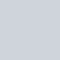# 看完這篇文章你會得到的成果圖# 前言【PyQt5】Day 5 – 開始來設計我們的 controller.py，改以「程式角度」來說明如何建立 PyQt 的系統

# 此篇文章的範例程式碼 github

https://github.com/howarder3/ironman2021_PyQt5_photoshop/tree/main/day17_roi_drawer

# 導入 OpenCV 作為繪圖引擎

• 如下圖：(點圖可放大)# UI 設計部份 (UI.py)

Qlabel 只能顯示就不能複製了。

*「顯示資訊用，不會改動 – Ratio ROI」：label_info_ratio_roi
*「顯示資訊用，不會改動 – Real ROI」：label_info_real_roi
*「依比例表示的 ROI 顯示欄位」：text_ratio_roi
*「依實際圖片座標表示的 ROI 顯示欄位」：text_real_roi## 轉換成 UI.py

### 轉換 day17.ui -> UI.py

``````pyuic5 -x day17.ui -o UI.py
``````

## 執行看看 UI.py 畫面是否如同我們想像

• 看看我們製作出來的介面
``````python UI.py
``````# controller 設計部份 (controller.py)

## 從 UI.py 中找出物件名稱

*「顯示資訊用，不會改動 – Ratio ROI」：label_info_ratio_roi
*「顯示資訊用，不會改動 – Real ROI」：label_info_real_roi
*「依比例表示的 ROI 顯示欄位」：text_ratio_roi
*「依實際圖片座標表示的 ROI 顯示欄位」：text_real_roi

## 老樣子，同 day13 的 scrollArea 說明，我們一樣需要刪除 scrollAreaWidgetContents 的部份

• 新增與調整的 scrollArea 片段
``````self.scrollArea = QtWidgets.QScrollArea(self.verticalLayoutWidget)
self.scrollArea.setWidgetResizable(True)
self.scrollArea.setObjectName("scrollArea")
# self.scrollAreaWidgetContents = QtWidgets.QWidget()
# self.scrollAreaWidgetContents.setGeometry(QtCore.QRect(0, 0, 937, 527))
# self.scrollAreaWidgetContents.setObjectName("scrollAreaWidgetContents")
# self.label_img = QtWidgets.QLabel(self.scrollAreaWidgetContents)
self.label_img = QtWidgets.QLabel() # 調整為只單純宣告
self.label_img.setGeometry(QtCore.QRect(0, 0, 941, 521))
self.label_img.setObjectName("label_img")
# self.scrollArea.setWidget(self.scrollAreaWidgetContents)
self.scrollArea.setWidget(self.label_img)
``````

## 取得名稱後，去修改控制部分

• controller.py：主要控制程式的部分
• img_controller.py：另外封裝專門處理圖片的部分
• opencv_engine.py：專門處理 OpenCV library 的引擎，基本上大部分圖像處理都靠他

### 專門處理圖像的引擎 opencv_engine.py

``````import cv2

class opencv_engine(object):

@staticmethod
def point_float_to_int(point):
return (int(point), int(point))

@staticmethod

@staticmethod
def draw_point(img, point=(0, 0), color = (0, 0, 255)): # red
point = opencv_engine.point_float_to_int(point)
print(f"get {point=}")
point_size = 1
thickness = 4
return cv2.circle(img, point, point_size, color, thickness)
``````

### 專門處理圖片的 img_controller.py

#### 導入 opencv_engine.py

(前者為 .py 檔名，後者為 class 名稱)

``````from opencv_engine import opencv_engine
``````

``````def read_file_and_init(self):
try:
self.origin_height, self.origin_width, self.origin_channel = self.origin_img.shape
except:
self.origin_height, self.origin_width, self.origin_channel = self.origin_img.shape
``````

#### 畫點 draw_point(self, point)

``````def draw_point(self, point):
# give me normalized point, i will help you to transform to origin cv image position
cv_image_x = point*self.origin_width
cv_image_y = point*self.origin_height
self.display_img = opencv_engine.draw_point(self.display_img, (cv_image_x, cv_image_y))
self.__update_img()

``````

#### 更新 roi 內的文字 __update_text_point_roi(self, point)

``````def __update_text_point_roi(self, point):
# give me normalized point, i will help you to transform to origin cv image position
cv_image_x = point*self.origin_width
cv_image_y = point*self.origin_height
self.ui.text_ratio_roi.append(f"[{point:.6f}, {point:.6f}]")
self.ui.text_real_roi.append(f"[{int(cv_image_x)}, {int(cv_image_y)}]")
``````

#### 修改傳回點座標的 get_clicked_position

``````def get_clicked_position(self, event):
x = event.pos().x()
y = event.pos().y()
self.__update_text_clicked_position(x, y)
norm_x = x/self.qpixmap.width()
norm_y = y/self.qpixmap.height()
self.draw_point((norm_x, norm_y))
self.__update_text_point_roi((norm_x, norm_y))
``````

## 修改並重新整理系統流程

### 目前的程式邏輯### 所以我們就把流程圖優化成下圖### 統一命名格式 set, update

• set 開頭的 function：可以外部呼叫，為修改事件的開頭
• __update 開頭的 function：皆為 private function，不可外部呼叫，只作為更新畫面使用

## 於是，這是最終修改後的 img_controller.py

``````from PyQt5 import QtCore
from PyQt5.QtGui import QImage, QPixmap

from opencv_engine import opencv_engine

class img_controller(object):
def __init__(self, img_path, ui):
self.img_path = img_path
self.ui = ui
self.ratio_value = 50

try:
self.origin_height, self.origin_width, self.origin_channel = self.origin_img.shape
except:
self.origin_height, self.origin_width, self.origin_channel = self.origin_img.shape

self.display_img = self.origin_img
self.__update_text_file_path()
self.ratio_value = 50 # re-init
self.__update_img()

def set_path(self, img_path):
self.img_path = img_path

def __update_img_ratio(self):
self.ratio_rate = pow(10, (self.ratio_value - 50)/50)
qpixmap_height = self.origin_height * self.ratio_rate
self.qpixmap = self.qpixmap.scaledToHeight(qpixmap_height)
self.__update_text_ratio()
self.__update_text_img_shape()

def __update_img(self):
bytesPerline = 3 * self.origin_width
qimg = QImage(self.display_img, self.origin_width, self.origin_height, bytesPerline, QImage.Format_RGB888).rgbSwapped()
self.qpixmap = QPixmap.fromImage(qimg)
self.__update_img_ratio()
self.ui.label_img.setPixmap(self.qpixmap)
self.ui.label_img.setAlignment(QtCore.Qt.AlignLeft | QtCore.Qt.AlignTop)
self.ui.label_img.mousePressEvent = self.set_clicked_position

def __update_text_file_path(self):
self.ui.label_file_name.setText(f"File path = {self.img_path}")

def __update_text_ratio(self):
self.ui.label_ratio.setText(f"{int(100*self.ratio_rate)} %")

def __update_text_img_shape(self):
current_text = f"Current img shape = ({self.qpixmap.width()}, {self.qpixmap.height()})"
origin_text = f"Origin img shape = ({self.origin_width}, {self.origin_height})"
self.ui.label_img_shape.setText(current_text+"\t"+origin_text)

def __update_text_clicked_position(self, x, y):
# give me qpixmap point
self.ui.label_click_pos.setText(f"Clicked postion = ({x}, {y})")
norm_x = x/self.qpixmap.width()
norm_y = y/self.qpixmap.height()
print(f"(x, y) = ({x}, {y}), normalized (x, y) = ({norm_x}, {norm_y})")
self.ui.label_norm_pos.setText(f"Normalized postion = ({norm_x:.3f}, {norm_y:.3f})")
self.ui.label_real_pos.setText(f"Real postion = ({int(norm_x*self.origin_width)}, {int(norm_y*self.origin_height)})")

def set_zoom_in(self):
self.ratio_value = max(0, self.ratio_value - 1)
self.__update_img()

def set_zoom_out(self):
self.ratio_value = min(100, self.ratio_value + 1)
self.__update_img()

def set_slider_value(self, value):
self.ratio_value = value
self.__update_img()

def set_clicked_position(self, event):
x = event.pos().x()
y = event.pos().y()
self.__update_text_clicked_position(x, y)
norm_x = x/self.qpixmap.width()
norm_y = y/self.qpixmap.height()
self.draw_point((norm_x, norm_y))
self.__update_text_point_roi((norm_x, norm_y))

def draw_point(self, point):
# give me normalized point, i will help you to transform to origin cv image position
cv_image_x = point*self.origin_width
cv_image_y = point*self.origin_height
self.display_img = opencv_engine.draw_point(self.display_img, (cv_image_x, cv_image_y))
self.__update_img()

def __update_text_point_roi(self, point):
# give me normalized point, i will help you to transform to origin cv image position
cv_image_x = point*self.origin_width
cv_image_y = point*self.origin_height
self.ui.text_ratio_roi.append(f"[{point:.6f}, {point:.6f}]")
self.ui.text_real_roi.append(f"[{int(cv_image_x)}, {int(cv_image_y)}]")
``````

## 執行結果

``````python start.py
``````# Reference